Definitions of Square Dance Calls and Concepts

Index -->  Plus  |  A1  |  A2  |  C1  |  C2  |  C3A  |  C3B  |  C4  |  NOL  |
Definitions (Text Only) -->  Plus  |  A1  |  A2  |  C1  |  C2  |  C3A  |  C3B  |  C4  |  NOL  |
 Find call:

\$BE,@Z\$J(B 4 \$B?M\$N(B (\$B\$=\$7\$F(B 8 \$B?M\$N(B) formation \$B\$+\$i(B.

Centers \$B\$O(B (\$BI,MW\$G\$"\$l\$P(B Touch \$B\$r\$7(B, \$B\$=\$7\$F(B) Cast Off 3/4 \$B\$r\$7(B, Ends \$B\$O(B 1/4 Right \$B\$r\$7\$^\$9(B.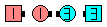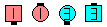Turnstyle\$B\$NA0(B \$B8e(B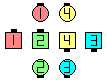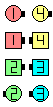Turnstyle\$B\$NA0(B \$B8e(B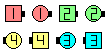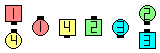Turnstyle\$B\$NA0(B \$B8e(B

\$BCm
• Cast Off 3/4 \$B\$O(B Touch 3/4, Right Arm Turn 3/4, Left Arm Turn 3/4, \$B\$^\$?\$O(B 'Push Cast' 3/4 \$B\$H\$J\$jF@\$^\$9(B.
• \$B%*%j%8%J%k\$NDj5A\$G\$O(B, Cast \$B\$O>o\$K(B "\$B1&(B" \$B\$N\$h\$&\$K=q\$+\$l\$F\$\$\$^\$7\$?(B.
•Choreography for TurnstyleComments? Questions? Suggestions?